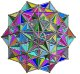# Math Is Fun Forum

Discussion about math, puzzles, games and fun.   Useful symbols: ÷ × ½ √ ∞ ≠ ≤ ≥ ≈ ⇒ ± ∈ Δ θ ∴ ∑ ∫  π  -¹ ² ³ °

You are not logged in.

## #1 2020-08-14 00:18:02

wintersolstice
Real MemberRegistered: 2009-06-06
Posts: 128

### Interesting way to express the Ackermann Function

There's a function (Ackermann Function) (though there are related functions)

Note: I had to improvise a bit with notation, plus I reused a few symbols (letters for variables) sorry if it's long and complicated, I also hope I've done it all correctly

defined as follows

rule 1

i.e.

rule 2

i.e.

and

otherwise

rule 3

I think I've come up with another way to express it

take

and

and use the different levels of arithmetic

level 0

(successor function)

level 1

(addition with B copies of 1)

level 2

(multiplication with B copies of A)

level 3

(exponentation with B copies of A)

level 4

(tetration with B copies of A)

and so on...

means level Y so

means A to B level Y of arithmetic

so based on this

with level 0 you do

Proof

which is

then take M=1

according to rule 2

according to rule 1

according to rule 3

according to rule 1

so you get the sequence

etc

compared to

etc

so

and

according to rule 2

according to the previous case

according to the previous case

creating this sequence

compared to

which means

and

m=3

according to rule 2

according to previous case

so with a first term of 5 and the operation multiply by 2 and then add 3

the formula will be of the form

multiply by 2 then add 3

and

so

and

so

so

now if L is used to mean a given level (where we know the formual works, and I've shown it works up to level 3) and LL means the next level

lets assume it works for a value of

first assumption

according to rule 2

and given the above rule

since

for any level

also given that

substitute

according to first assumption

to generalise (is a second assumption, though it works for 0 and 1)

so

substituting

and

with (P+3) 2's)

with (P+4) 2's)

given that

and so on

Why did the chicken cross the Mobius Band?
To get to the other ...um...!!!

Offline